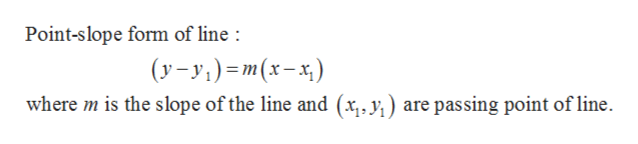# Graph the line that passes through the given point and has the given slope.P(1, 7);slope−2

Question

Graph the line that passes through the given point and has the given slope.

P(1, 7);
slope
−2

check_circle

Step 1

Given:

Step 2

Formlua used:help_outlineImage TranscriptionclosePoint-slope form of line (y-y) m(x-x) where m is the slope of the line and (x,, y,) are passing point of line fullscreen
Step 3

In this ca...

### Want to see the full answer?

See Solution

#### Want to see this answer and more?

Solutions are written by subject experts who are available 24/7. Questions are typically answered within 1 hour.*

See Solution
*Response times may vary by subject and question.
Tagged in

### Other